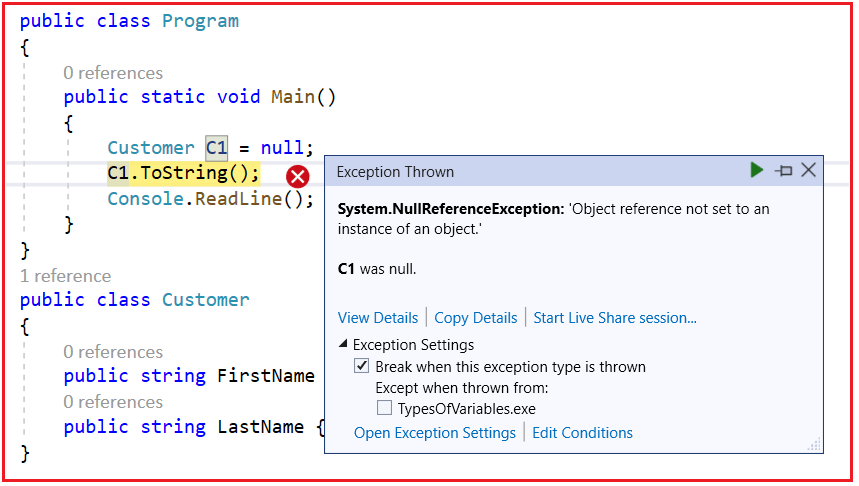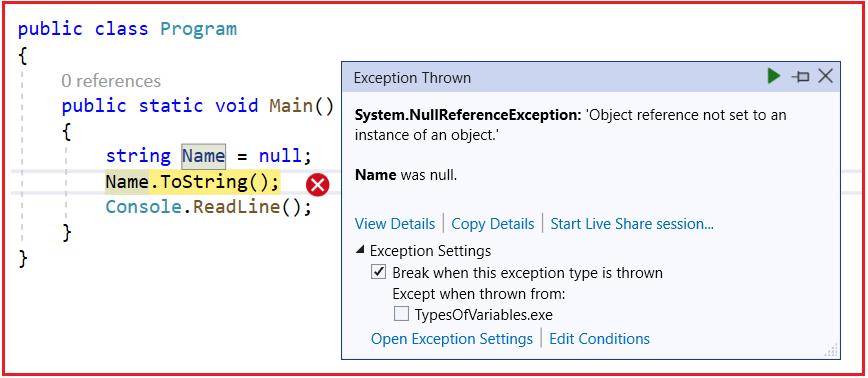# Difference Between Convert.ToString and ToString Method in c#

## Difference Between Convert.ToString and ToString Method in C#

In this article, I am going to discuss the Difference Between Convert.ToString and ToString Method in C# with Examples. Please read our previous article before proceeding to this article where we discussed why and how to override the Equals Method in C# with examples.

##### Convert.ToString and ToString Method in C#

Both these methods are used to convert a value to a string. The difference is Convert.ToString() method handles null whereas the ToString() doesn’t handle null in C#.

In C# if you declare a string variable and if you don’t assign any value to that variable, then by default that variable takes a null value. In such a case, if you use the ToString() method then your program will throw the Null Reference Exception. On the other hand, if you use the Convert.ToString() method then your program will not throw an exception.

###### Let us understand the Difference Between these two methods with an example.
```using System;
namespace UnderstandingToStringMethod
{
public class Program
{
public static void Main()
{
Customer C1 = null;
C1.ToString();
}
}
public class Customer
{
public string FirstName { get; set; }
public string LastName { get; set; }
}
}
```

When you run the application, it will give you the following errorThis is because the ToString() method in C# expects the object to be not NULL on which it is invoking. In our example object, C1 is Null and we are invoking the ToString() on the NULL object, so it gives NULL Reference exception.

##### Let see another example.
```using System;
namespace UnderstandingToStringMethod
{
public class Program
{
public static void Main()
{
String Name = null;
Name.ToString();
}
}
}
```

When we execute the above program, it also gives us the same NULL Reference Exception as shown below.This is because the Name variable is Null and we are invoking the ToString() method. Let see what happens when we use the Convert.Tostring() method with the above two examples.

```using System;
namespace UnderstandingObjectClassMethods
{
public class Program
{
public static void Main()
{
Customer C1 = null;
Convert.ToString(C1);

String Name = null;
Convert.ToString(Name);

Console.WriteLine("No Error");
}
}
public class Customer
{
public string FirstName { get; set; }
public string LastName { get; set; }
}
}```

Now, with the above changes, run the application and it should be executed without any error. So in short, the Convert.ToString() method handles null, while the ToString() method doesn’t handle the Null and throws an exception. So it’s always a good programming practice to use the Convert.ToString() method which will take care of the Null values and it is also safe to use.

### 3 thoughts on “Difference Between Convert.ToString and ToString Method in c#”

1.Mohamed Hassan

Then, When do we use .ToString() method rather than the Convert.ToString()?

1..ToString() method should be used when you are certain that user will enter data into the field that you are trying to convert. When there is uncertainty, you should use Convert.ToString()

2.Well explained
But I have a question….
suppose we have a class with some properties defined using get, set
public class Test
{
public string? name {get; set;}
}
–Now if I make a object of this class and apply Convert.ToString() directly on it —– it will throw exception why?
Test obj = new Test()
{
name = null
}

Console.WriteLine(Convert.ToString( obj.name )) // will throw exception why?